Courses

Revision Notes - Solutions Notes | Study NEET Revision Notes - NEET

NEET: Revision Notes - Solutions Notes | Study NEET Revision Notes - NEET

The document Revision Notes - Solutions Notes | Study NEET Revision Notes - NEET is a part of the NEET Course NEET Revision Notes.
All you need of NEET at this link: NEET

A solution is a homogeneous mixture of two (or more) substances, the composition of
which may vary between certain limits. A solution consisting of two components is called binary solution. The component which is present in large quantity is called solvent and the component which is small in quantity is called solute. If both components are in same physical state.

Type of Solutions:
All the three states of matter (gas, liquid or solid) may behave either as solvent or solute. Depending on the state of solute or solvent, mainly there may be the following seven types of binary solutions.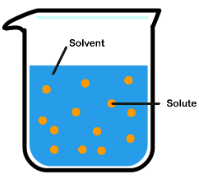S.No. Solute Solvent Example 1 Gas Gas Air 2 Gas Liquid Aerated water (CO2 + H2O) 3 Gas Solid Hydrogen in palladium 4 Liquid Liquid Alcohol in water, benzene in toluene 5 Liquid Solid Mercury in zinc amalgam 6 Liquid Gas CO2 dissolved in water 7 Solid Liquid Sugar in water, common salt in water 8 Solid Gas Smoke 9 Solid Solid Various alloys

VAPOUR PRESSURE

• The pressure exerted by vapours over the liquid surface at equilibrium is called vapour pressure of the liquid.
• If solute is non volatile solid or liquid the vapour pressure of solution is equal to partial vapour pressure of solvent in the solution.
• If the solute is volatile solid or liquid, then vapour pressure will be equal to the sum of partial vapour pressure of solute and that of solvent.

RAOULT'S LAW

“The partial vapour pressure of any component in the solution is directly proportional to its mole fraction”.
For a binary solution of two components A and B,
P = P°XA
PB = P°XB
Where,

• P0A  = vapour pressure of component A in pure state.
• P = vapour pressure of component A in the solution.
• P0B  = vapour pressure of component B in pure state.
• PB  = vapour pressure of component B in the solution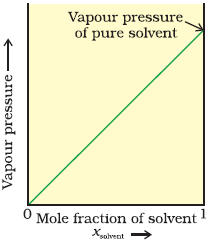Limitations of Raoult’s Law:

• Raoult’s law is applicable only to very dilute solutions.
• It is applicable to solutions containing non-volatile solute only.
• It is not applicable to solutes which dissociate or associate in a particular solution

Raoult’s Law in Combination with Dalton’s Law of Partial Pressure:
PT = XA P0A + XB P0B = P0B  + (P0-P0B) XA
Where
PT = Total Vapour Pressure of the Solution.

IDEAL AND NON-IDEAL SOLUTIONS
Ideal Solutions:
An ideal solution is the solution in which each component obeys Raoult’s law under all conditions of temperatures and concentrations.

Properties of Ideal solutions:
(i) ΔHmixing = 0, i.e. no heat should be absorbed or evolved during mixing
(ii) ΔVmixing  = 0, i.e. no expansion or contraction on mixing
(iii) Intermolecular attractive forces between the A-A and B-B are nearly equal to those between A-B.
Examples: Ethyl chloride and Ethyl bromide, n–hexane and n–heptane , CCland SiCl4

Non-Ideal Solutions:
When a solution does not obey Raoult’s law over the entire range of concentration, then it is called non-ideal solution.
For non ideal solutions,
(i) ΔHmixing  ≠ 0
(ii) ΔVmixing ≠ 0
Here we may have two cases
1. Positive Deviation:
(i) Solvent-Solute(A-B) type of force is weaker than Solute-Solute(B-B) & Solvent-Solvent(A-A) forces.
(ii) The vapour pressure is higher than predicted by the law.
(iii) ΔHmixing > 0
(iv) ΔVmixing > 0
Examples: Ethanol and Acetone, Carbon disulphide and Acetone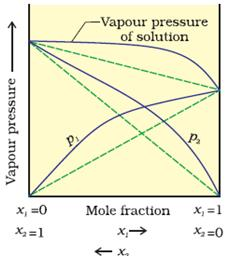2. Negative Deviation:
(i) Solvent-Solute(A-B) type of force is stronger than the other two.
(ii) The vapour pressure is lower than predicted by the law.
(iii) ΔHmix < 0
(iv) ΔVmix  < 0
Examples: Phenol and Aniline, Chloroform and Acetone
etc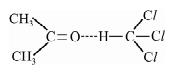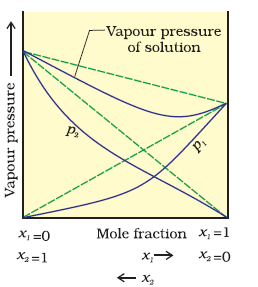COLLIGATIVE PROPERTIES
The properties of dilute solutions which depend only on number particles of solute present in the solution and not on their identity are called colligative properties (denoting depending upon collection).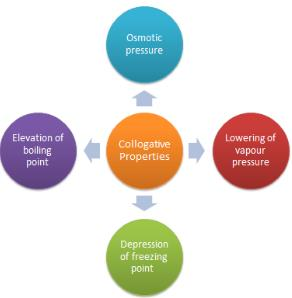Lowering of Vapour Pressure by a Non-Volatile Solute
The relative lowering of vapour pressure of a solution containing a non-volatile solute is equal to the mole fraction of the solute present in the solution.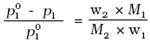Here w1 and w2 are the masses and M1 and M2 are the molar masses of the solvent and solute respectively.
Elevation of Boiling Point by a Non-Volatile Solute :
Since the addition of a non-volatile solute lowers the vapour pressure of the solvent, the vapour pressure of a solution is always lower than that of the pure solvent, and hence it must be heated to a higher temperature to make its vapour pressure equal to atmospheric pressure.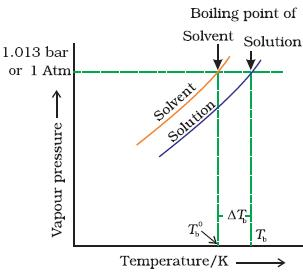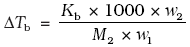where M1 = molecular weight of solute and w2 and w1 are weights of solute and solvent
Kb:
It is defined as the elevation in boiling point when the molality of the solution is unity.
The unit of Kb is K kg mol–1
Determination of Kb: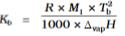where: R = Gas constant (8.314 JK/mol),
Tf = Freezing temperature in K,
M1 = Molar mass of solvent in Kg/mol,
ΔvapH = Enthalpy of vapourisation of solvent in J/mol.
Depression of Freezing Point by a Non-Volatile Solute: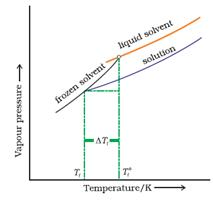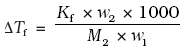where M1 = molecular weight of solute and wand w1 are weights of solute and solvent
Kf:
It is defined as the depression in freezing point when the molality of the solution is unity. The unit of Kf is K kg mol-1
Determination of Kf: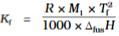where : R = gas constant (8.314 JK/mol),
Tf = freezing temperature in K,
M1 = Molar mass of solvent in Kg/mol,
ΔfusH = enthalpy of fusion of solvent in J/kg

Osmosis and Osmotic Pressure:

• Osmosis: The phenomenon of the passage of pure solvent from a region of lower concentration (of the solution) to a region of its higher concentration through a semi-permeable membrane.
• Osmotic Pressure: Excess pressure which must be applied to a solution in order to prevent flow of solvent into the solution through the semi-permeable membrane.

πV = nRT
where
π = Osmotic pressure
V = volume of solution
n = no. of moles of solute that is dissolved
R = Gas constant
T = Absolute temperature

Isotonic Solutions: Two solutions having same osmotic pressure at a given
temperature are called isotonic solutions.
The solution with lower concentration or lower osmotic pressure is known as “Hypotonic” with respect to more concentrated solution.
The solution with higher concentration or higher osmotic pressure is known as “Hypertonic” with respect to dilute solution.

ABNORMAL MOLECULAR WEIGHT AND VAN'T HOFF FACTOR
Van't Hoff Factor:
Van't Hoff, in order to account for all abnormal cases introduced a factor i known as the Van't Hoff factor, such that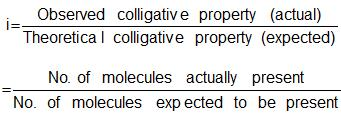Degree of Association:
The fraction of the total number of molecules which combine to form bigger molecule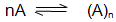Let a be the degree of association, then,
The number of unassociated moles = 1 - α
The number of associated moles = α/n
Total number of effective moles = 1- α + α /n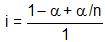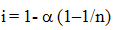Obviously,  i < 1
Degree of Dissociation:
The fraction of the total number of molecules which dissociates in the solution, that is, breaks into simpler molecules or ions.

KCl ↔  K+ + Cl-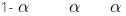Thus, the total number of moles after dissociation  = 1 - α + α + α = 1 + α
Hence, i = (1 + α)/1
i = 1 + α  = 1+ (2–1)α
In general, i = 1+ (n–1)α,
Where, n = number of particles ( ions) formed  after dissociation
From the above formula, it is clear that  i > 1

The document Revision Notes - Solutions Notes | Study NEET Revision Notes - NEET is a part of the NEET Course NEET Revision Notes.
All you need of NEET at this link: NEETUse Code STAYHOME200 and get INR 200 additional OFF Use Coupon Code

Top Courses for NEET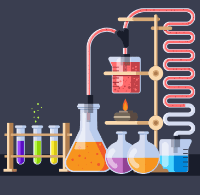NEET Revision Notes

291 docs

Top Courses for NEETHow to Prepare for NEET

Read our guide to prepare for NEET which is created by Toppers & the best Teachers

Track your progress, build streaks, highlight & save important lessons and more!

,

,

,

,

,

,

,

,

,

,

,

,

,

,

,

,

,

,

,

,

,

;